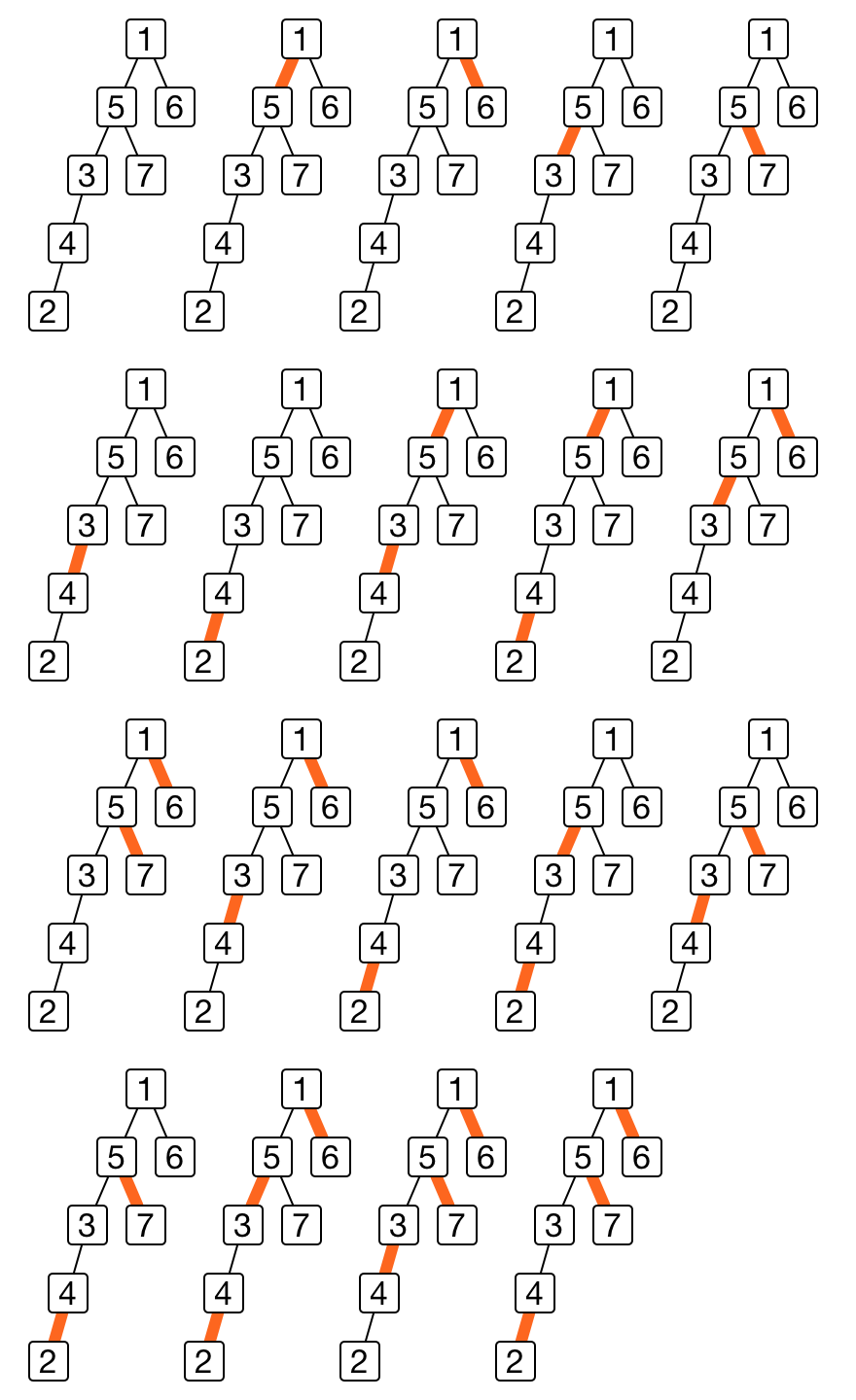Code Submission Evaluation System Login

# E4590 2018 2

 Start: 2018-09-22 13:00:00 End: 2018-09-22 16:00:00

CSES - E4590 2018 2 - Tree matchingCSES - Tree matching

## Tree matching

 Time limit: 1.00 s Memory limit: 128 MB

You are given a binary tree with $n$ nodes numbered $1, 2, 3, \ldots, n$. The root of the tree is node $1$. Your task is to determine how many ways there are to pair connected nodes so that each node is paired up with at most one another node.

As the number of ways may be large, output the result modulo $10^9+7$.

Input
The first line of the input contains a single number, $n$, the number of nodes in the tree.

Next $n$ lines each contain two integers, $l_i$ and $r_i$, describing the left and right child of $i^{th}$ node. $l_i$ is the index of left child of $i^{th}$ node and $r_i$ is the index of right child of $i^{th}$ node. Index $0$ means that no node is connected as a child.

Output
A single line, result modulo $10^9+7$.

Limits
• $1 \le n \le 10^6$
Example

Input:
7 5 6 0 0 4 0 2 0 3 7 0 0 0 0

Output:
19

Explanation: